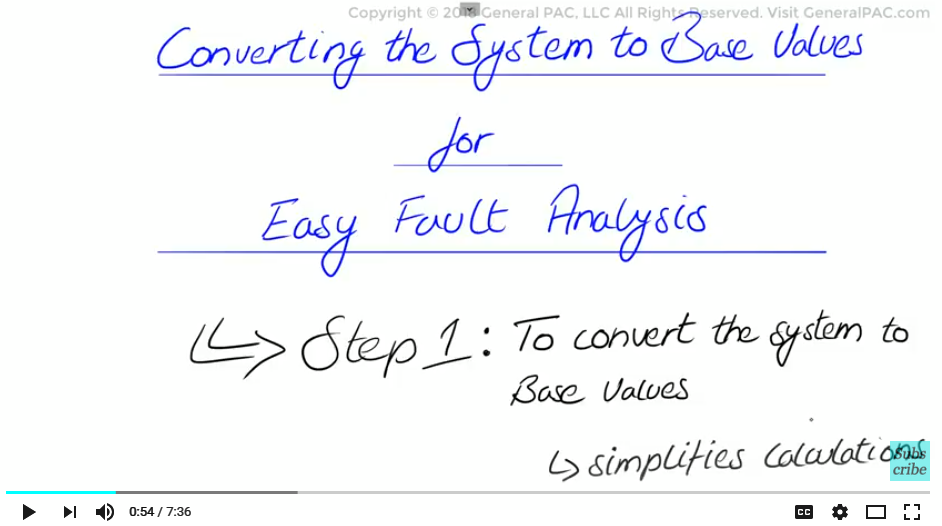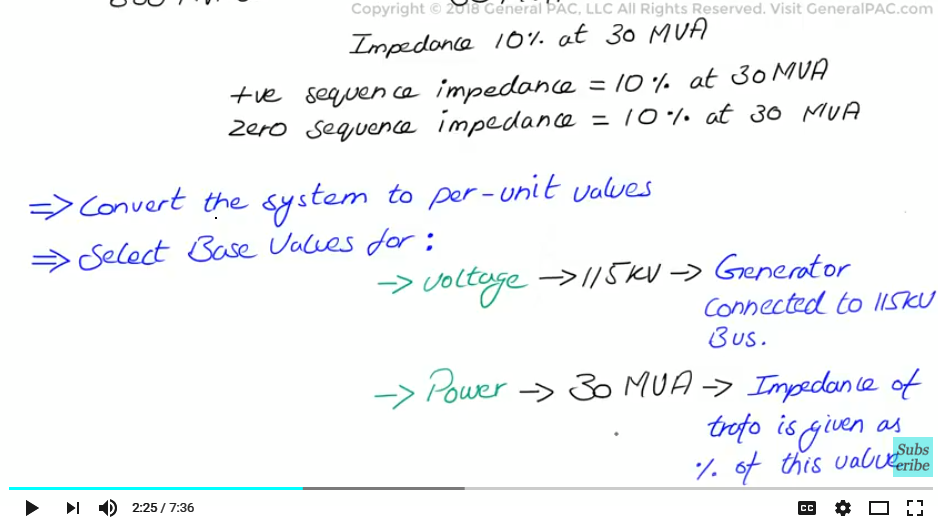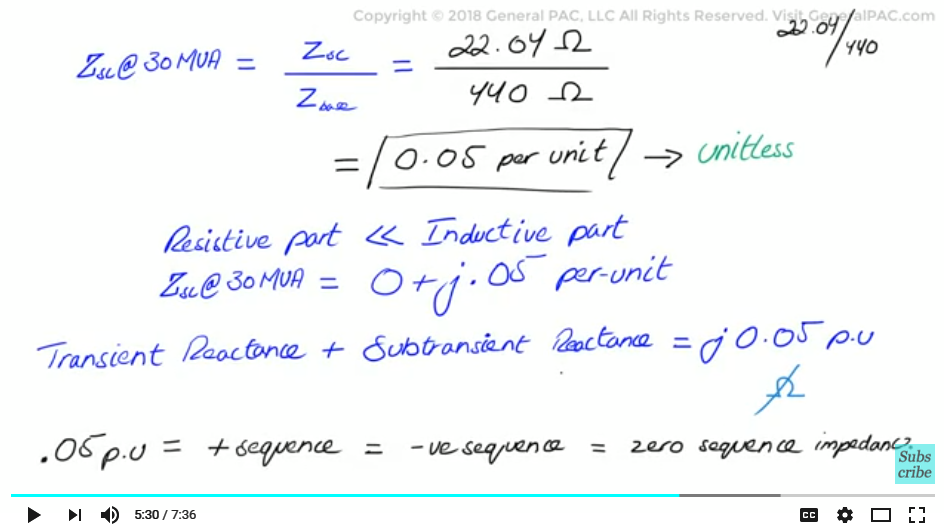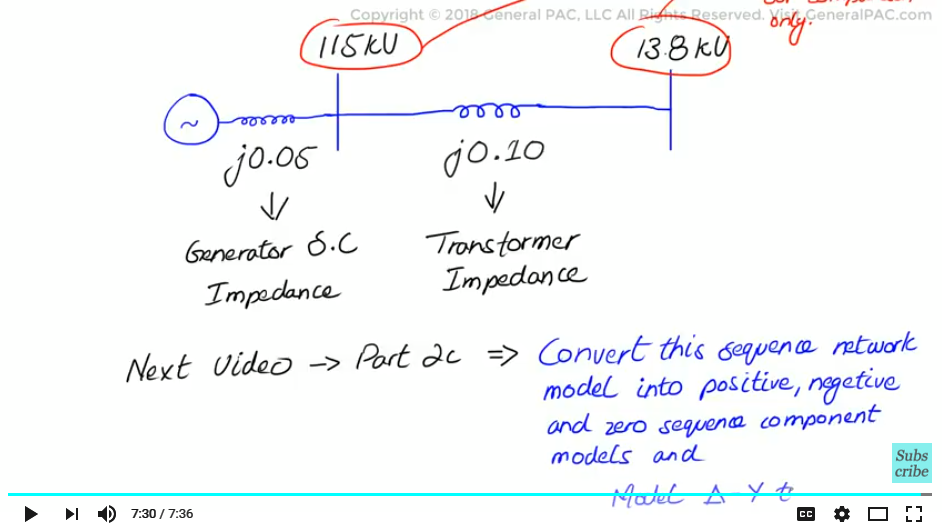This video was brought to you by GeneralPAC.com, making power systems Intuitive, Open and Free for Everyone, Everywhere. Consider subscribing and supporting through patreon.com/GeneralPAC. This is a mechanism for you to support us financially so we can continue making high quality power system video tutorials. Our corporate sponsor for this topic is AllumiaX.com from Seattle, Washington. Contact them for industrial and commercial power system studies.

Fault analysis in power systems Part 2b: Converting the System to Base Values for Easy Fault Analysis. Now this is the third video of the series on fault analysis in power systems. In this video we will proceed to step number 1 of our fault calculation process and that is to convert the system to base values and we will show how this simplifies our calculations considerably.Now let’s introduce this example, we have a three phase synchronous generator which has a short circuit capability of 600 MVA SC that is connected to a 115 KV bus. We also have a delta-wye transformer rated at 30 MVA which connects the 115 KV bus to the 13.8 KV bus and has an impedance of 10% at 30 MVA. Now we will add additional information by saying that the transformer positive sequence impedance is 10% at 30 MVA and we will also claim that the zero sequence impedance is also 10 % at 30 MVA.

Now remember our objective here is to convert the system impedances into per unit values or per unit equivalents and that will simplify our fault calculations quite a bit down the road. Now for this we need to select base values for voltage and power that is the first step, so we will select 115 KV as our base voltage because the generator is connected to the 115 KV bus and we will select 30 MVA as the base power, base value for power because the impedance of the transformer is given as a percentage of this value which is 10 % at 30 MVA. Now we will see the advantage of this down the road, but let’s continue.

Now given the base value of voltage and power, we can now calculate base value for the impedance which is really simple, the impedance base or the Z base will equal the base voltage divided by the base value of power. That is just our old formula P = V^2 divided by R. We are just rearranging it to solve for R. In this case Z. so Z base is equal to the KV base squared divided by MVA base which is equal to 115 KV^2 divided by 30 MVA which equals 440.83 ohms. The 440 Ohms now being the base value of impedance.Now let’s derive the generator per unit impedance as well as the transformer per unit impedance. We will start with the generator per unit impedance derivation, we must find the short circuit impedance value of the generator and convert it to the per unit value. Now for this we go back to our legacy formula which is P = V2 divided by R, re arranging it to solve for R. In this case it’s the Z short circuit equals to 115 kV squared divided by 600 MVA that is equal to 22.04 ohms. To convert that to the per unit value we simply divide this 22.04 ohms by the base impedance value of 440 ohms that we calculated earlier so the per unit value of the short circuit impedance at 30 MVA is equal to short circuit impedance divided by the base value of impedance that is 22.04 ohms divided by 440.83 ohms which conveniently gives us .05 per unit, remember per unit is a unit less value because practically speaking the resistive part is very small as compared to the inductive part of the generator, we write this as Z short circuit at 30 MVA is equal to 0 + j0.05 per unit. Now just to simplify this a little more and to clarify it we are going to assume that the transient reactance of the generator as well as the sub transient reactance is going to equal this j0.05 per unit for simplicity. Remember we are forgetting the ohms, we are dropping the ohms and now we are talking about per unit values.

Furthermore we are going to assume that 0.05 per unit is the same as the generators positive sequence impedance, negative sequence impedance as well as zero sequence impedance. So we are assuming that the generator’s sequence impedances are going to equal j0.05 per unit.Now lets move on to the transformer per unit impedance derivation. The impedance was given at 10 % at 30 MVA for both the positive sequence and the zero sequence impedance since we selected 30 MVA as our base earlier it just comes out to be that the positive sequence impedance of the transformer is equal to 0 + j0.10 per unit. Again we assume that the zero sequence impedance is going to be the same so the zero sequence impedance of the transformer is also going to be 0 + j0.10 per unit. Now the 0.10 per unit as seen in the equation is assuming that it is all reactive, so we are going to assume that we don’t have a resistive part of the impedance.

Now once we draw the values in a per unit converted system its basically the generator symbol which is attached to an inductor which we are gonna call j.05 impedance which is the generator impedance that is connected to the 115 KV bus, and which is connected to another inductor which we will call j.10 impedance that is the transformer impedance connected to the 13.8 KV bus, now the that we have shown, these are just for display purposes they are not really representing the voltages as again we are using per unit values to remove the voltages from our calculations. So that is just for our own comprehension.

Now in the next video in Part 2c we will show how to convert this sequence network model into positive negative and zero sequence components and model the delta wye transformer within the sequence network diagram. Thank you#### Greetings from the GeneralPAC Team!

We make high-quality Power Systems Video Tutorials on complex topics that are free and open to everyone!  Thank you so much for supporting us through Patreon so can continue doing good and valuable work.

What is Patreon and why do we use it?

Patreon is a fantastic portal that allows our fans and community to make monthly contribution (like Netflix subscription) so we can continue creating high-quality power systems video tutorials. In return, you get access to incredible perks like voting on future topics, getting your questions answered, access to VIP Q/A webinars with the creators of GeneralPAC, and much more! We THANK YOU for supporting us

Why do we need your support?

An incredible amount of time and effort is needed to develop high-quality video tutorials. Each video (Part 1 for example) takes approximately 10 hours to complete which includes learning the concept ourselves, brainstorming creative ways to teach and explain the concepts, writing the script, audio recording, video recording, and editing. It's no wonder why Hundreds-of-Thousands of people have watched, liked, subscribed, and left positive comments on Youtube channel. Your support truly makes all the difference.